Παρασκευή, 4 Νοεμβρίου 2016

Harmonic Mean in Geometry Ο αρμονικός μέσος στη γεωμετρία !

Harmonic Mean in Geometry

Harmonic Mean in Geometry

The harmonic mean H(a,b) of two positive numbers a and b is defined as

H(a,b)=21a+1b,
and equivalently

2H(a,b)=1a+1b,
or else

H(a,b)=2aba+b.
It makes a most dramatic appearance in the problem of average speed, but also pops up in various geometric circumstances. Below I collect a few examples.

1. Let AGB be a semicircle and AC=a, BC=b.If CGAB and CHOG then GH=H(a,b).

2. In a trapezoid ABCD, let EF be the line parallel to the bases AB and CD through the point of intersection of the diagonals AC and BD: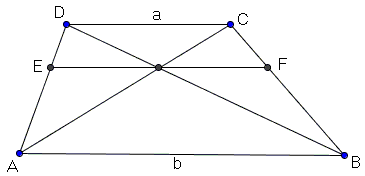If CD=a and AB=b, then EF=H(a,b).

It is worth observing that the point of intersection of the diagonals divides EF into two equal parts, which leads to another common example:

3. In a 1877 sangaku from the Hyogo prefecture, AE and BD are both perpendicular to AB. P is the intersection of AD and BE, and CPAB.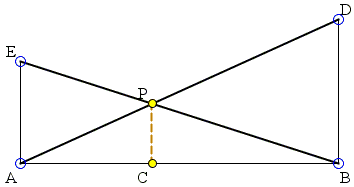If AE=a and BD=b, then CP=2H(a,b).

4. The same motif reemerges in the See-Saw Lemma where AE, BF, and EF are tangent (the latter at X) to the semicircle AXB.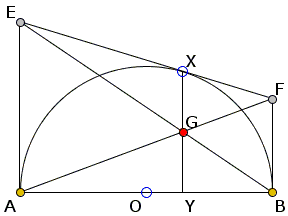If AE=a and BF=b, then XY=H(a,b).

5. Vladimir Nikolin from Serbia has observed appearance of the harmonic mean in his Rhombus Lemma.Let AD be the bisector of A in ΔABC, BD||AC and CD||AB. Then ABDC is a rhombus and its side p is half of the harmonic mean of sides b=AC and b=AB.

Choosing the angle at A to be right leads to a special case where the rhombus becomes a square.

6. Let a square be inscribed into a right triangle as in the diagram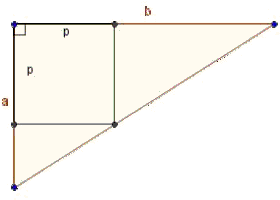Then, if a and b are the legs of the triangle and p the side of the square, 1p=1a+1b. In other words, p=12H(a,b).

7. As a consequence of the Rhombus Lemma there is a simple way of constructing the harmonic ratio: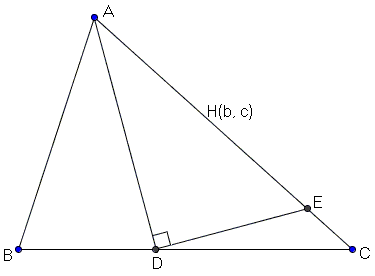In ΔABC, with AB=c and AC=b, draw AD - the bisector of A. At D erect a perpendicular to AD and let E be its intersection with either AB or AC. Then AE=H(b,c).• The Means

• Averages, Arithmetic and Harmonic Means

• Expectation

• The Size of a Class: Two Viewpoints

• Averages of divisors of a given integer

• Family Statistics: an Interactive Gadget

• Averages in a sequence

• Arithmetic and Geometric Means

• Geometric Meaning of the Geometric Mean

• A Mathematical Rabbit out of an Algebraic Hat

• AM-GM Inequality

• The Mean Property of the Mean